# MP Board Class 10th Maths Solutions Chapter 5 Arithmetic Progressions Ex 5.2

In this article, we will share MP Board Class 10th Maths Book Solutions Chapter 5 Arithmetic Progressions Ex 5.2 Pdf, These solutions are solved subject experts from the latest edition books.

## MP Board Class 10th Maths Solutions Chapter 5 Arithmetic Progressions Ex 5.2Question 1.
Fill in the blanks in the following table, given that a is the first term, d the common difference and an the nth term of the A.P.
Solution: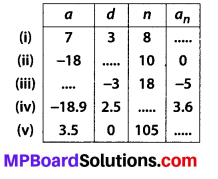Solution:
(i) an = a + (n- 1)d
a8 = 7 + (8 – 1)3 = 7 + 7 × 3 = 7 + 21
⇒ a8 = 28

(ii) an = a + (n – 1)d
⇒ a10 = -18 + (10 – 1)7 ⇒ 0 = -18 + 9d
⇒ 9d = 18 ⇒ d = $$\frac{18}{9}=2$$
∴ d = 2

(iii) an = a + (n – 1)d
⇒ -5 = a + (18 – 1) × (-3)
⇒ -5 = a + 17 × (-3)
⇒ -5 = a – 51 ⇒ a = -5 + 51 = 46
Thus, a = 46

(iv) an = a + (n – 1)d
⇒ 3.6 = -18.9 + (n – 1) × 2.5
⇒ (n – 1) × 2.5 = 3.6 + 18.9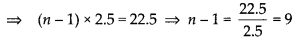⇒ n = 9 + 1 = 10
Thus, n = 10

(v) an = a + (n- 1)d
⇒ an = 3.5 + (105 – 1) × 0
⇒ an = 3.5 + 104 × 0 ⇒ an = 3.5 + 0 = 3.5
Thus, an = 3.5

Question 2.
Choose the correct choice in the following and justify:
(i) 30th term of the AP: 10,7,4, , is, ….,
(A) 97
(B) 77
(C) -77
(D) -87

(ii) 11th term of the AP: -3, $$-\frac{1}{2}$$, 2, …. ,is
(A) 28
(B) 22
(C) -38
(D) -48$$\frac{1}{2}$$
Solution:
(i) (C): Here, a = 10, n = 30
∵ T10 = a + (n – 1)d and d = 7 – 10 = -3
∴ T30 = 10 + (30 – 1) × (-3)
⇒ T30 = 10 + 29 × (-3)
⇒ T30 = 10 – 87 = -77

(ii) (B): Here, a = -3, n = 11 andCommon difference calculator is the difference between two successive terms of an arithmetic progression.

Question 3.
In the following APs, find the missing terms in the boxes: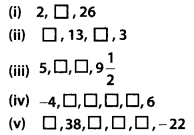Solution:
(i) Here, a = 2, T3 = 26
Let common difference = d
∴ Tn = a + (n- 1 )d
⇒ T3 = 2 + (3 – 1)d
⇒ 26 = 2 + 2 d
⇒ 2d = 26 – 2 = 24(ii) Let the first term = a
and common difference = d
Here, T2 = 13 and T4 = 3
T2 = a + d = 13, T4 = a + 3d = 3
T1 – T2 = (a + 3d) – (a + d) = 3 – 13(iii)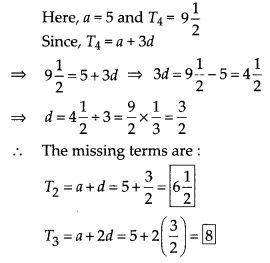(iv) Here, a = – 4, T6 = 6
∵ Tn = a + (n -1 )d
T6 = – 4 + (6 – 1)d ⇒ 6 = -4 + 5d ⇒ 5d = 6 + 4 = d = 10 – 5 = 2
T2 = a + d = -4 + 2 =-2
T3 = a + 2d = -4 + 2(2) = 0
T4 = a + 3d = -4 + 3(2) = 2
T5 = a + 4d = -4 + 4(2) = 4(v) Here, T2 = 38 and T6 = -22
∴ T2 = a + d = 38, T6 = a + 5d = -22
⇒ T6 – T2 = a + 5d – (a + d) = -22 – 38 -60
⇒ 4d = -60 ⇒ d = $$\frac{-60}{4}$$ = -15
a + d = 38 ⇒ a + (-15) = 38
⇒ a = 38 + 15 = 53
Now,
T3 = a + 2d = 53 + 2(-15) = 53 – 30 = 23
T4 = a + 3d = 53 + 3(-15) = 53 – 45 = 8
T5 = a + 4d = 53 + 4(-15) = 53 – 60 = -7
Thus missing terms areQuestion 4.
Which term of the AP: 3, 8, 13, 18, is 78?
Solution:
Let the nth term be 78
Here, a = 3 ⇒ T1 = 3 and T2 = 8
∴ d = T2 – T1 = 8 – 3 = 5
And, Tn = a + (n- 1 )d
⇒ 78 = 3 + (n – 1) × 5 ⇒ 78 – 3 = (n -1) × 5
⇒ 75 = (n – 1) × 5 ⇒ (n – 1) = 75 ÷ 5 = 15
⇒ n = 15 + 1 = 16
Thus, 78 is the 16th term of the given AP.

Question 5.
Find the number of terms in each of the following APs:
(i) 7,13,19, …….. ,205
(ii) 18, $$15 \frac{1}{2}$$, 13, …… ,-47
Solution:
(i) Here, a = 7,d = 13 – 7 = 6
Let total number of terms be n.
∴ Tn = 205
Now, Tn = a + (n – 1) ×d
= 7 + (n – 1) × 6 = 205
⇒ (n – 1) × 6 = 205 – 7 = 198
⇒ n – 1 = $$\frac{198}{6}=33$$
∴ n = 33 + 1 = 34
Thus, the required number of terms is 34.

(ii)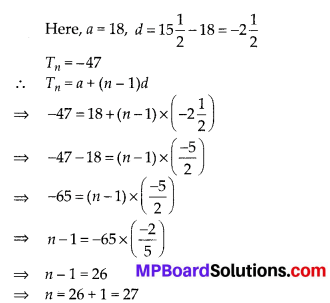Thus, the required number of terms is 27.Question 6.
Check whether -150 is a term of the AP:
11, 8, 5, 2…
Solution:
For the given AP,
we have a = 11, d = 8 -11 = -3
Let -150 be the nth term of the given AP
∴ Tn = a + (n – 1 )d
⇒ -150 = 11 + (n – 1) × (-3)
⇒ -150 – 11 = (n – 1) × (-3)
⇒ -161 = (n – 1) ⇒ (-3)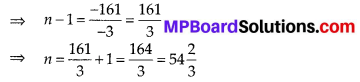But n should be a positive integer.
Thus, -150 is not a term of the given APQuestion 7.
Find the 31st term of an AP whose 11th term is 38 and the 16th term is 73.
Solution:
Here, T11 = 38 and T16 = 73
Let the first term = a and the common difference = d.
Tn = a + (n – 1 )d
Then, Tn = a + (11 – 1)d = 38
⇒ a + 10d = 38 …(1)
and T16 = a + (16 – 1)d = 73
⇒ a + 15d = 73 …(2)
Subtracting (1) from (2), we get
(a + 15d) – (a + 10d) = 73 – 38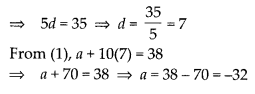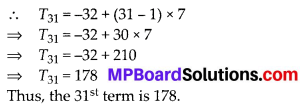Question 8.
An AP consists of 50 terms of which 3rd term is 12 and the last term is 106. Find the 29th term.
Solution:
Here, n = 50, T3 = 12, Tn = 106
⇒ T50 = 106
Let the first term = a and the common difference = d
Tn = a + (n – 1 )d
T3 = a + 2d = 12 …(1)
T50 = a + 49d = 106 …(2)
Subtracting (1) from (2), we getQuestion 9.
If the 3rd and the 9th terms of an AP are 4 and -8 respectively, which term of this AP is zero?
Solution:
Here, T3 = 4 and T9 = -8
Tn = a + (n – 1)d
T3 = a + 2d = 4 …. (1)
T9 = a + 8d = – 8 …. (2)
Subtracting (1) from (2), we getQuestion 10.
The 17th term of an AP exceeds its 10th term by 7. Find the common difference.
Solution:
Let a be the first term and d the common difference of the given AP
Now, using n = a + (n – 1 )d, we have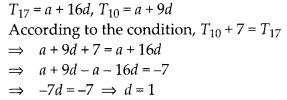Thus, the common difference is 1.Question 11.
Which term of the AP : 3, 15, 27, 39,… will be 132 more than its 54th term?
Solution:
Here, a = 3, d = 15 – 3 = 12
Using Tn = a + (n – 1 )d, we getThus, 132 more than 54th term is the 65th term.

Question 12.
Two APs have the same common difference. The difference between their 100th terms is 100, what is the difference between their 1000th terms?
Solution:
Let for the 1st AP, the first term = a and common difference = dAnd for the 2nd AP, the first term = a and common difference = d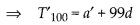According to the condition,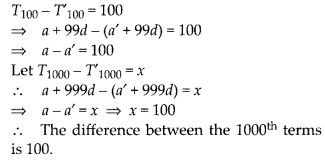Question 13.
How many three-digit numbers are divisible by 7?
Solution:
The first three-digit number divisible by 7 is 105.
The last such three-digit number divisible by 7 is 994.
∴ The AP is 105,112,119, ,994
Let n be the required number of terms Here, a = 105, d = 7 and n = 994Thus, 128 numbers of 3-digits are divisible by 7.

Question 14.
How many multiples of 4 lie between 10 and 250?
Solution:
The first multiple of 4 beyond 10 is 12.
The multiple of 4 just below 250 is 248.Thus, the required number of terms is 60.Question 15.
For what value of n, are the nth terms of two APs: 63,65,67 … and 3,10,17, …. equal?
Solution:Thus, the 13th terms of the two given AP’s are equal.

Question 16.
Determine the AP whose third term is 16 and the 7th term exceeds the 5th term by 12.
Solution:
Let the first term = a and the common difference = d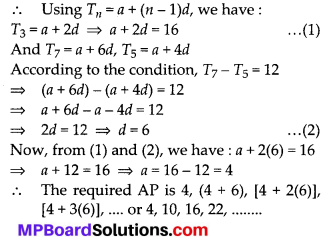Question 17.
Find the 20th term from the last term of the AP : 3, 8, 13, …, 253.
Solution:
We have, the last term (l) = 253
Here, d = 8 – 3 = 5
Since, the nth term before the last term is given by l – (n – 1 )d
We have 20th term from the last term = l – (20 – 1) × 5 = 253 – 19 × 5 = 253 – 95 = 158

Question 18.
The sum of the 4th and 8th terms of an AP is 24 and the sum of the 6th and 10th terms is 44. Find the first three terms of the AP.
Solution:
Let the first term = a and the common difference = dQuestion 19.
Subba Rao started work in 1995 at an annual salary of ₹ 5000 and received an increment of ? 200 each year. In which year did his income reach ₹ 7000?
Solution:
Here, a = ₹ 5000 and d = ₹ 200
Let, in the nth year Subba Rao gets ₹ 7000.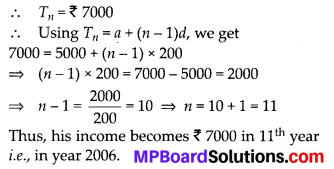Question 20.
Ramkali saved ₹ 5 in the first week of a year and then increased her weekly savings by ₹ 1.75. If in the nth week, her weekly savings become ₹ 20.75, find n.
Solution:
Here, a = ₹ 5 and d = ₹ 1.75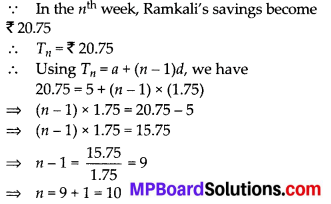Thus, the required number of weeks is 10.